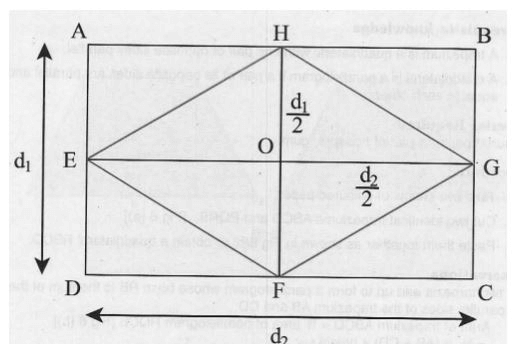# Theory - To show that the Area of Rhombus is half the Product of its Diagonals, Math, Class 9 Class 9 Notes | EduRev

## Class 9 : Theory - To show that the Area of Rhombus is half the Product of its Diagonals, Math, Class 9 Class 9 Notes | EduRev

The document Theory - To show that the Area of Rhombus is half the Product of its Diagonals, Math, Class 9 Class 9 Notes | EduRev is a part of the Class 9 Course Mathematics (Maths) Class 9.
All you need of Class 9 at this link: Class 9

As performed in the real lab:

Materials Required:

Coloured papers, sketch pens, geometry box, a pair of scissors, fevicol and eraser.

Procedure:

1.Draw □ ABCD with length d2 and breadth d1 units on a coloured  paper.

2. Mark points E, F, G and H as midpoints of the sides AD, DC, CB and BA respectively of sides of the □ ABCD.

3. Join HF and EG. Mark their intersection as point O. Fold the rectangle ABCD along EG and HF dividing the □ ABCD into four congruent rectangles, namely OEAH, OEDF, OFCG and OGBH.

4. Divide each of the four rectangles into two congruent triangles by drawing their respective diagonals.As performed in the simulator:

1. Provide dimensions of a rectangle i.e. length and breadth in the input box to draw the rectangle.

2. Mark midpoint of each side of the rectangle by clicking on the midpoint of each side.

3. Connect midpoints of opposite sides.

4. Connect midpoints of adjacent sides to form a rhombus.

5. Cut four triangles that are in the corner of rectangle ABCD using "Cut Traingle" button.

6. Place each triangle to the position mentioned in "Instructions".

Observation:

1. As the small rectangles are congruent, their diagonals EH, HG, GF, FE are equal. Thus EHGF is a rhombus.

2. In the rectangle AHOE, triangles AHE and EHO are congruent and hence equal in area.

3. Thus area of the right triangle EOH is half the area of the rectangle AEOH and similarly, the area of right triangles HOG, GOF, FOE are half the area of the rectangles HBGO, OGCF and FOED respectively.

4. Thus the area of rhombus EFGH =  (1/2 X Area of rectangle AEOH) + (1/2 X Area of rectangle HBGO) + (1/2 X Area of rectangle OGCF) + (1/2 X Area of rectangle FOED)

= 1/2 X ( Area of rectangle AEOH + Area of rectangle HBGO + Area of rectangle OGCF + Area of rectangle FOED)

= 1/2 x Area of rectangle ABCD

= 1/2 x d1 x d2

= 1/2 X HF X EG

= 1/2 x product of diagonals of rhombus EFGH

Result:

Area of rhombus is half the product of its diagonals.

Offer running on EduRev: Apply code STAYHOME200 to get INR 200 off on our premium plan EduRev Infinity!

## Mathematics (Maths) Class 9

190 videos|233 docs|82 tests

,

,

,

,

,

,

,

,

,

,

,

,

,

,

,

,

,

,

,

,

,

,

,

,

,

,

,

;